Conjugation of Trees and Random Maps

Gilles Schaeffer

LaBRI, Université Bordeaux I

Algorithms Seminar

February 1, 1999

[summary by Alain Denise]

A properly typeset version of this document is available in postscript and in pdf.

If some fonts do not look right on your screen, this might be fixed by configuring your browser (see the documentation here).

Abstract
We present a general scheme to generate uniformly at random planar maps of various kinds. Our algorithms rely on a combinatorial and bijective approach: we encode the planar maps by some classes of particular trees. This encoding is the basis of efficient random generators of maps of the most simple classes of planar maps. For more complex structures, we proceed by using a new general probabilistic scheme, that we call extraction/rejection algorithm.

## 1   Introduction

A planar map is an embedding of a graph in the plane, considered up to continuous deformations of the plane. Maps are allowed to have multiple edges and loops; otherwise there are called simple maps. The maps we consider are rooted: there is an oriented edge, called the root.

We present here two families of algorithms for the random generation of rooted planar maps. The first one applies to the few classes of maps which are counted by multiplicative closed formulas. Here are two examples from [2, 5]:
Number of planar maps with n edges:
2
n+2
3n
2n+1
æ
è
 2n+1 n
ö
ø
,
Number of bipartite cubic maps with 3n vertices:
3
n+2
2n-1
2n+1
æ
è
 2n+1 n
ö
ø
.
Our approach consists in finding new bijections between these maps and some particular families of trees. More precisely, there exists, for each family of maps as above, a family of trees such that
#{maps} =
#{free leaves}
#{leaves}
· #{trees},
and the bijection is given by the closure of the trees (terms like free leaves and closure will be explained below). Then the generation scheme is the following: i) generate uniformly at random a tree, by known efficient algorithms; ii) ``decode'' the tree to get its associated map. Table 1 gives the basic families that benefit from this approach, leading to linear generation algorithms. The method is illustrated in Section 2 on the family of bipartite cubic maps.

main family intermediate family underlying trees
general quartic (all degree 4) binary
bipartite (or Eulerian) bipartite cubic binary
m-constellations m-Eulerian general
non separable quartic without 2-cocycle ternary
loopless triangulations non separable cubic ternary

Table 1: Maps (with n edges, loops and multiple edges allowed) generated in linear time

However, a number of families do not present simple formulas as above. In these cases we use a new method: extraction/rejection. It combines the usual rejection principle with composition schemes that are common for maps. See in Table 2 the new families reached with this new approach, which is presented in Section 3.

maps simple smooth no leaf 2-c non separ. 3-c 4-c
all ok ok ok ok ok ok no
bipartite ok no ok ok ok no no
triangulations ok - - - - ok ok

Table 2: Some extra properties reachable via extraction/rejection. (Non separable means loopless 2-connected, smooth means without vertices of degree 2)

## 2   Bijective Approach

We present here the method through the example of bipartite cubic maps: maps whose vertices have degree 3 and are colored in black or white in such a way that no edge joins two vertices of the same color (Figure 1).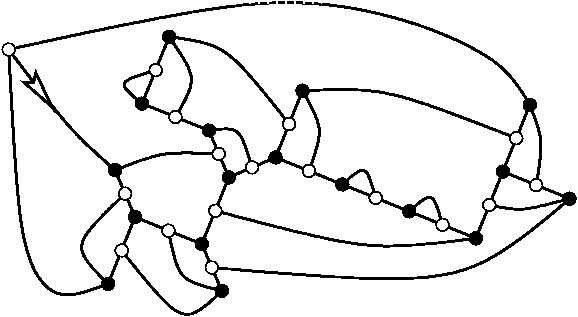Figure 1: A bipartite cubic map with 17 × 2 vertices

These maps are in bijection with blossom trees, as we will see below. A planted plane binary tree is a plane binary tree whose root has degree one. Figure 2 presents such a tree (the leaves are omitted in the figure, except the root leaf at the left). A blossom tree is a particular tree which can be constructed from a planted plane tree as follows: in the middle of each internal edge, we put a white-colored vertex with a bud on one of the two sides, as in Figure 3.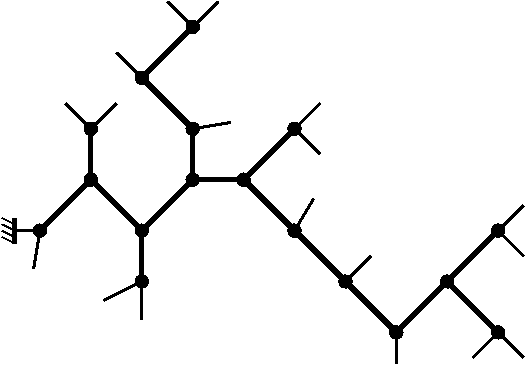Figure 2: A planted plane tree with 17 internal nodes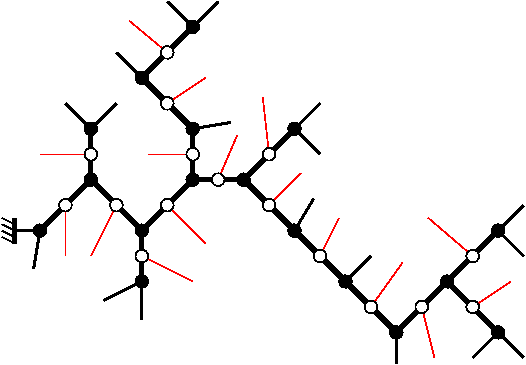Figure 3: A blossom tree

Now we call partial closure of a blossom tree the structure obtained after the following treatment: turn anti-clockwise around the tree, and join each bud to the nearest leaf so that no crossing occurs. (In this way, buds and leaves can be seen as a system of nested parentheses.) See in Figure 4 the partial closure of the blossom tree of Figure 3. Since there are 3 more leaves than buds in any blossom tree, there are necessarily 3 leaves which remain alone; we call them single leaves. We say that a blossom tree is balanced if its root is a single leaf in its partial closure. Finally the complete closure of a blossom tree is obtained by joining the three single leaves to a new vertex and rooting the resulting map towards the root leaf (Figure 5). This gives the map of Figure 1.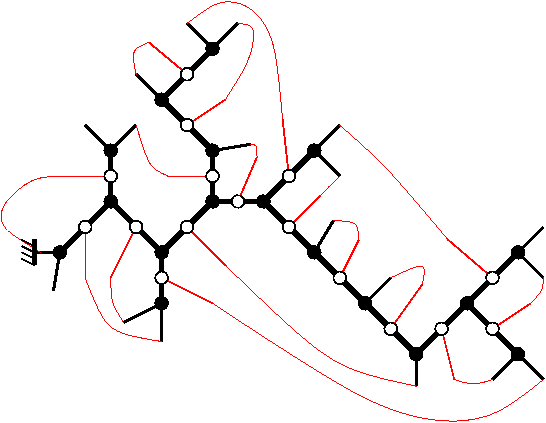Figure 4: Partial closure of the blossom tree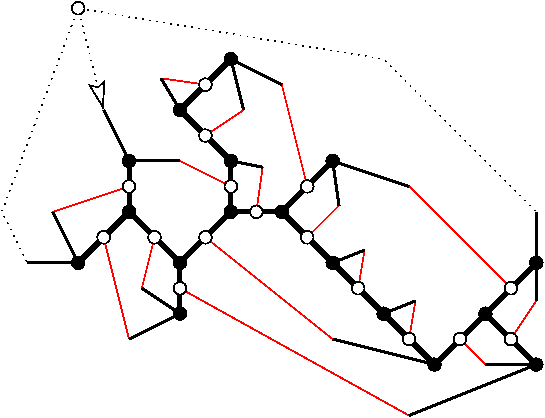Figure 5: Complete closure of the blossom tree

Theorem 1   The complete closure defines a one-to-one correspondence between balanced blossom trees with n nodes and bipartite cubic maps with 3n edges.

Now here is the way to generate uniformly at random a bipartite cubic map with 3n edges:
1. generate uniformly at random a planted binary tree with n nodes; this can be done in linear complexity with well known algorithms (see  for example);
2. toss a coin independently for each edge to place buds;
3. choose with probability 1/3 one of the three possible balanced blossom trees and achieve the complete closure.

## 3   Extraction/Rejection Algorithms

Suppose that we have to generate uniformly at random a bicolored triangulation like the one in Figure 6, with a given number of faces.

By duality, this is equivalent to generate a bipartite cubic map as the one in Figure 7: each black (resp. white) vertex in the map gives a black (resp. white) triangle in the triangulation, each face gives a vertex.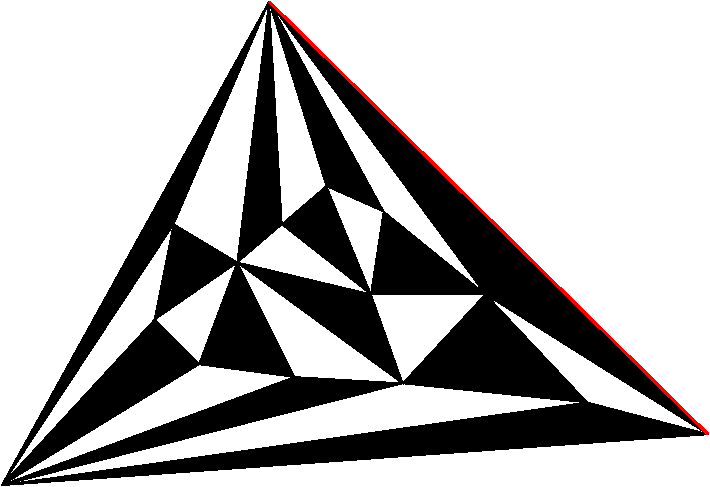Figure 6: A bicolored triangulation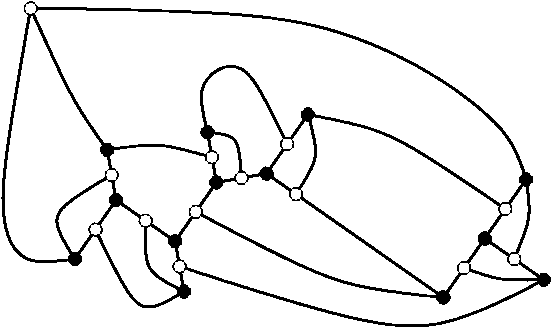Figure 7: A bipartite cubic map without separator

But the map must be without separator, i.e., without configuration like the one on the left of Figure 8; otherwise the associated triangulation might present multiple edges, like in the right of the figure.

A natural idea would be to use a rejection algorithm in order to generate maps without separators: draw uniformly at random bipartite cubic maps until we get a map without separator. Unfortunately, bipartite cubic maps without separators are very rare, so this approach is practically intractable. So we need another idea. Here is the extraction/rejection method:
1. Generate uniformly at random a bipartite cubic map with the algorithm of Section 2;
2. remove the possible separators (we get what we call the core of the map);
3. construct the triangulation from the core by duality.
An important problem appears here: if we generate a map with n vertices and remove the separators, the resulting map is likely to have less than n vertices! To fix this problem, we will generate maps with too many vertices: we can prove, using asymptotic analysis, that drawing bipartite cubic maps with m=3n vertices gives, with a good probability, cores having n vertices. This leads to an algorithm with complexity O(n5/3).

This approach can be generalized for a number of families, as seen in Table 2. Details are given in [3, 4].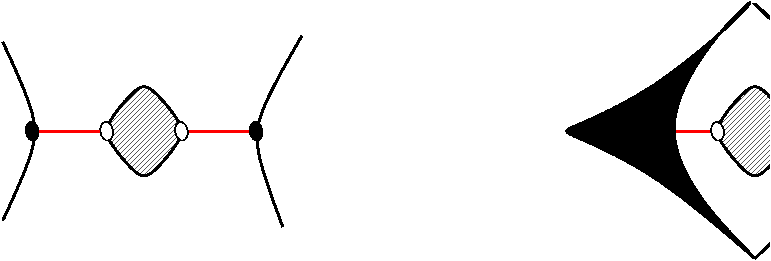Figure 8: A separator in a bipartite cubic map and its result by duality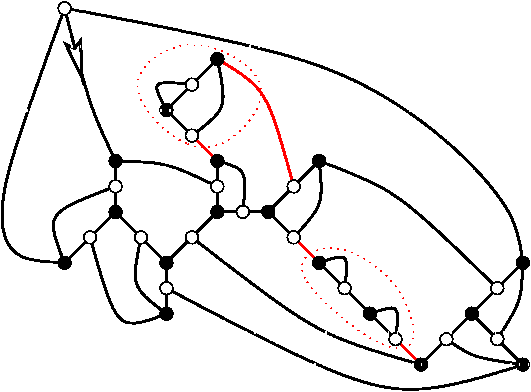Figure 9: A map with separators

## References


Alonso (Laurent) and Schott (René). -- Random generation of trees. -- Kluwer academic publishers, 1995.


Brown (W. G.) and Tutte (W. T.). -- On the enumeration of rooted non-separable planar maps. Canadian Journal of Mathematics, vol. 16, 1964, pp. 572--577.


Schaeffer (Gilles). -- Conjugaison d'arbres et cartes combinatoires aléatoires. -- PhD thesis, Université Bordeaux I, 1998.


Schaeffer (Gilles). -- Random sampling of large planar maps and convex polyhedra. In Proceedings of STOC'99. -- Atlanta, 1999.


Tutte (W. T.). -- On the enumeration of planar maps. Bulletin of the American Mathematical Society, vol. 74, 1968, pp. 64--74.

This document was translated from LATEX by HEVEA.